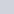# 15 – B.6. Top-Level Planning and Analysis

• This topic has 1 reply, 2 voices, and was last updated 1 month, 2 weeks ago byLynn Roden.
• Creator
Topic
• #238272Saed Albahri
Participant

Hi,

page 281, attached depreciation (how I can calculate the depreciation % prorated among COGS, inventory, and SG&A ?

page 283, note payable, how is the additional fund calculated (adjustment) ?

thank you

Viewing 1 replies (of 1 total)
• Author
Replies
• #238284Lynn Roden
HOCK international

Hello Saed Albahri,

The percentages for 20X1 are calculated by dividing each type of actual depreciation by total actual depreciation recorded. Total depreciation was 4,409. Depreciation in COGS was 3,160, which is 71.7% of 4,409 (3,160 / 4,409). Depreciation in Inventory was 840, which is 19.1% of 4,409 (840 / 4,409). Depreciation in SG&A expense was 409, which is 9.2% of 4,409 (409 / 4,409).

The additional funds needed is the difference between the “required assets” (93,060) and the “specified sources of financing” (88,442). If a forecasted balance sheet were being prepared for 20X2, the 4,618 in additional funds needed would be what would be required to make Total Assets equal Total Liabilities and Equity.

Lynn

Viewing 1 replies (of 1 total)
• You must be logged in to reply to this topic.